# TexturizePolygons

Make 2D and 3D graphics with specified polygons and corresponding textures

 ResourceFunction["TexturizePolygons"][ps,tsp] makes a 2D or 3D plot for the list of polygons ps using the textures specification tsp. ResourceFunction["TexturizePolygons"][ps] uses a random texture.

## Details and Options

ResourceFunction["TexturizePolygons"] takes all options of Graphics, Graphics3D, and ResourceFunction["RandomMandala"].
In addition to a list of polygons, the first argument to ResourceFunction["TexturizePolygons"] can also be a string name for an entity in .
The second argument can be an association, a list of 2D graphics, Automatic or Random.
If the second argument is an association then it is expected to map numbers of vertices to textures.
If the second argument is a list of 2D graphics then those graphics are converted to textures.
In general it is more aesthetically pleasing when polygons with the same number of vertices have the same textures.
The option "Granularity" can be used to control texture mapping. If its value is "NumberOfVertices" then polygons with the same number of vertices have the same textures. If its value is "Polygon" then each polygon has its own texture (according to the texture specification.)
The option “VertexTextureCoordinatesFunction” is used to tune the assignment of texture coordinates to polygon vertices.

## Examples

### Basic Examples

Here polygons for the dodecahedron are given a random texture:

 In:=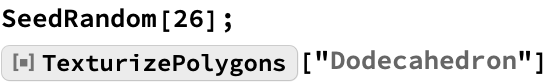Out=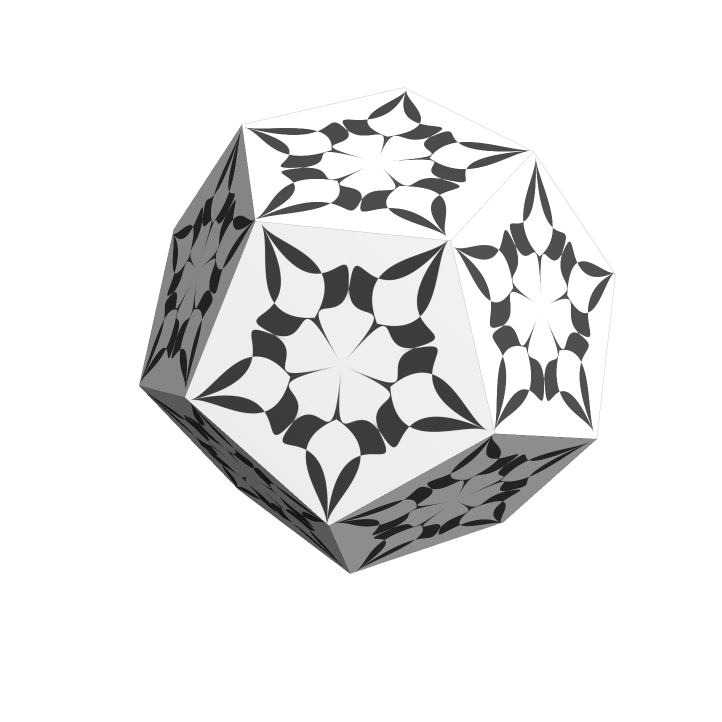Here is an unfolded texturized dodecahedron:

 In:=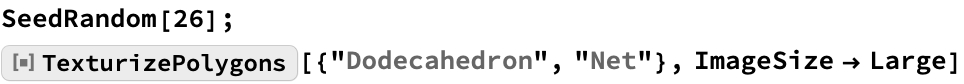Out=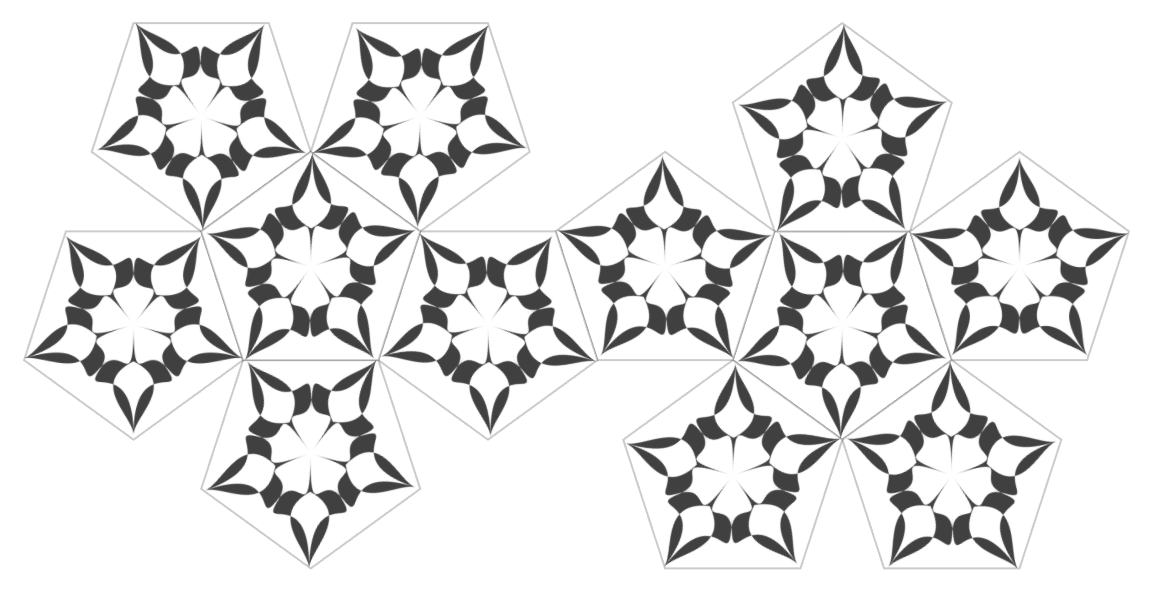### Scope

The PolyhedronData properties "Net" and "Faces" can be used together with polyhedron names; 2D and 3D graphics are respectively produced:

 In:=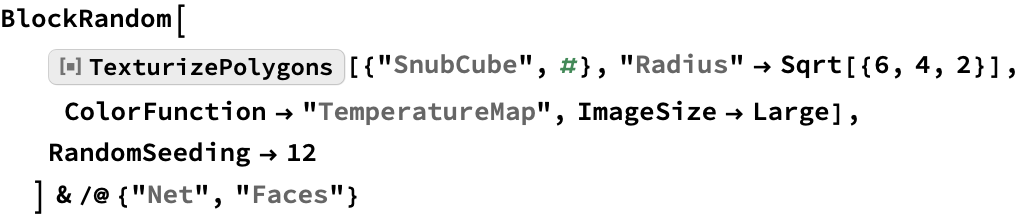Out=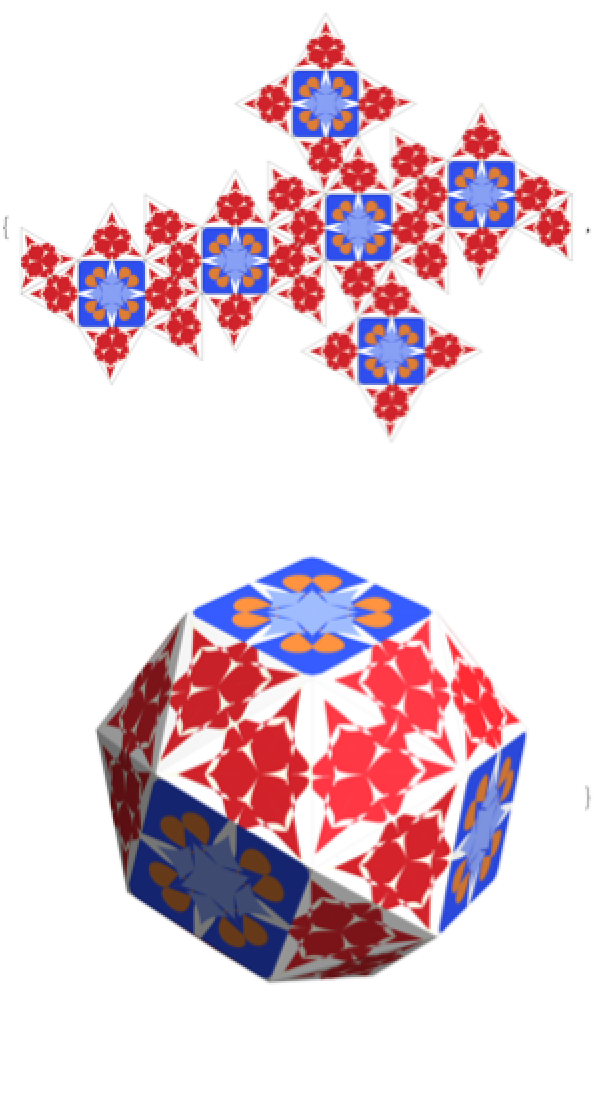Here is an application over a list of random 2D polygons, translated and rotated for better comprehension:

 In:=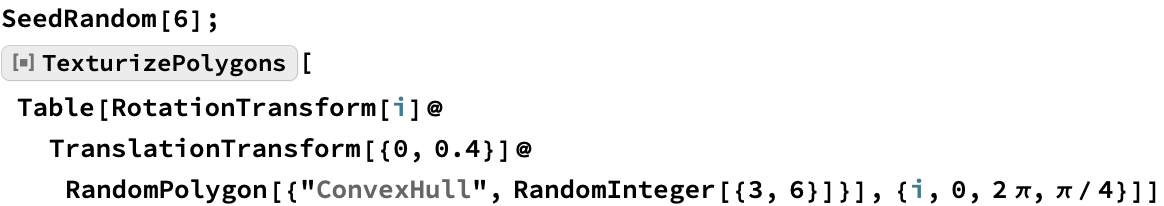Out=